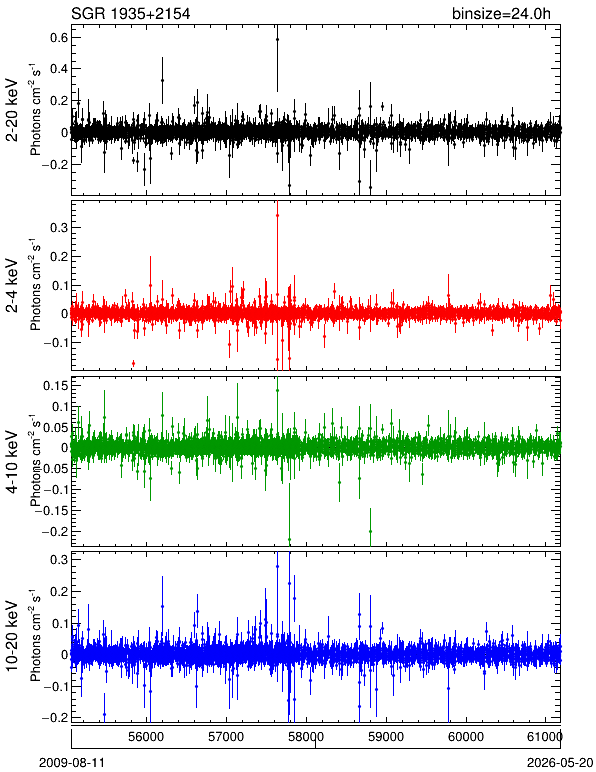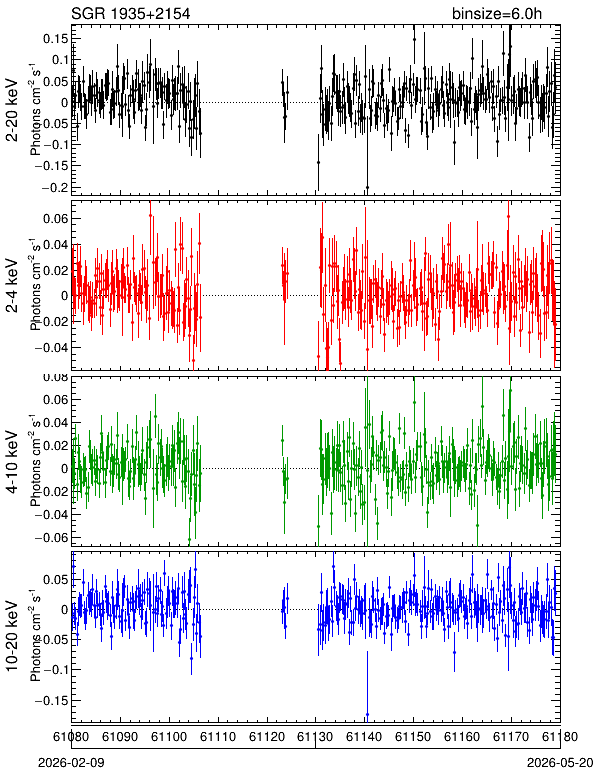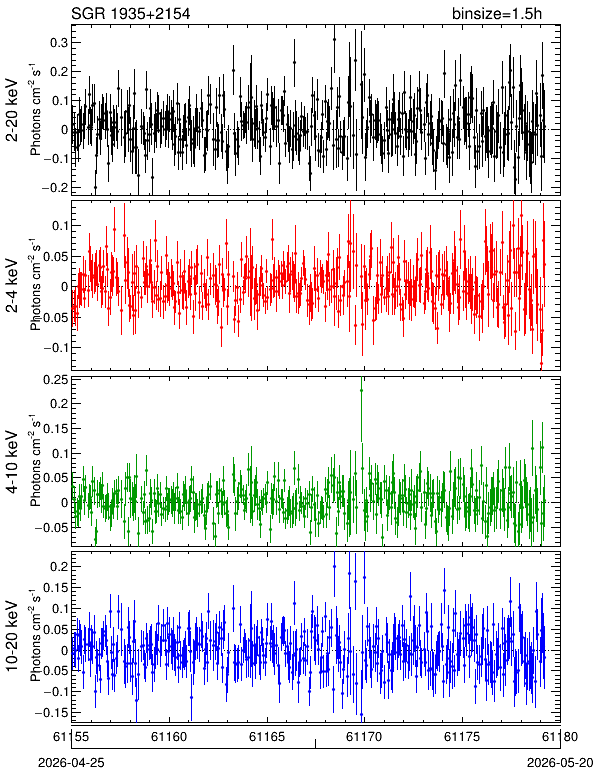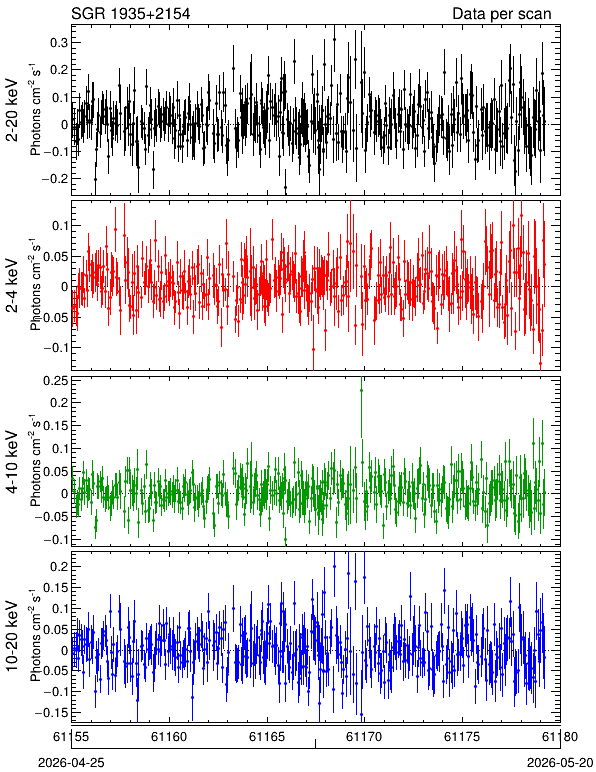# SGR 1935+2154

J2000 (RA, Dec) = (293.732, 21.897)
Galactic (L, B) = (57.247, 0.819)
Data processed until UT Date = 2023-06-01 (MJD = 60096)

### • Light Curve

• The error bars represent only the statistical uncertainties at 1-sigma.
• Data of GSCs with broken anodes (GSC_#3, GSC_#6) are excluded.
HV Binsize = 24.0 h Binsize = 6.0 h Binsize = 1.5 h Scan
Good
GSCsPDF QDP CSV FITS PDF QDP CSV FITS PDF QDP CSV FITS PDF QDP CSV FITS